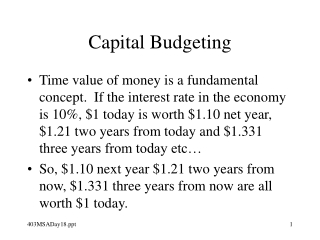DownloadDownload PresentationCapital Budgeting

# Capital Budgeting

Télécharger la présentation## Capital Budgeting

- - - - - - - - - - - - - - - - - - - - - - - - - - - E N D - - - - - - - - - - - - - - - - - - - - - - - - - - -
##### Presentation Transcript

1. Capital Budgeting • Time value of money is a fundamental concept. If the interest rate in the economy is 10%, \$1 today is worth \$1.10 net year, \$1.21 two years from today and \$1.331 three years from today etc… • So, \$1.10 next year \$1.21 two years from now, \$1.331 three years from now are all worth \$1 today.

2. Capital Budgeting • Now if I am to get \$1.1 next year, \$1.21 the year after and \$1.331 the third year, what should I be willing to pay for the right to this stream of cash flows assuming that my only other alternative is to put the money in a bank account and get 10% interest? • Ans: \$3, why? • Each year’s cash inflow is worth a dollar today.

3. Capital Budgeting • If someone wants to sell me this investment for \$2.90, my NPV (net present value) of the project is _____ • Ans: 10cents. How computed? • The cash inflows are worth \$3 in today’s dollars, the outflows are \$2.90 in today’s dollars, so the NPV (always in current dollars) is Cash Inflows – Cash Outflows = \$0.10.

4. Capital Budgeting • The basic equation of compound interest is shown on p. 96: PV(1+r)n = FV • (1+r)n is called the “factor” • To get the present value of a stream of cash inflows divide each future inflow amount by the factor for that year (this is called deflating the FV) and add all the deflated inflows … this is the formula on p.97.

5. Capital Budgeting • To get the present value of a stream of cash outflows compute the sum of the deflated cash outflows. • To compute NPV of a project subtract PV(outflows) from PV(Inflows). • To do the computations by hand you can use special formulas for perpetuities and annuities. We will ignore this. • For this course, you should know how to do the computations using a financial calculator.

6. Capital Budgeting • To correctly compute project NPV: • Use cash flows not accounting earnings. Remember to adjust for depreciation (and the tax consequences of depreciation) … see pp 110-11. • Exclude interest costs from relevant cash flows else you will be double-counting. • Include investment in working capital in funding requirements and discount the required additional investments at future points. • Include opportunity costs, ignore sunk costs.

7. Capital Budgeting • Besides NPV, people also use • Payback period • Time taken to earn back the original investment (there is no discounting of any cash flows in this method). • This method may be useful when long-term cash flows are uncertain. However it can lead to serious mistakes in project selection since it ignores the “tail” of cash flows beyond the recovery of the initial amount. • In effect this method is very conservative and not a good first choice to use.

8. Capital Budgeting • Other measures: • ROI (you know this) • IRR • The discount rate that makes the NPV of the project zero. You can compute this on any financial calculator. • There may be multiple IRRs for a single project, so this method can produce confusing answers. • IRR may be used to prioritize among projects when capital is limited: select projects with the highest IRR till you have used up all your capital. • However, this rule can be seriously misleading as well since it assumes that all cash inflows can be invested at the project’s internal rate of return which is an unrealistic assumption.

9. Capital Budgeting • Moral: • Use NPV as the first step in evaluating projects. • If capital is in short supply, try and find the best mix of projects to take using simulation rather than use some arbitrary short-cuts (IRR etc.). • Look at payback period as a second step, especially if the projects are otherwise comparable (in magnitude of investment, life of cash flows). If strategic flexibility in the firm’s investment base matters, payback period is a healthy tool in spite of serious theoretical deficiencies. • In other words, be careful of using only NPV because cash flows in the far future are hard to predict.